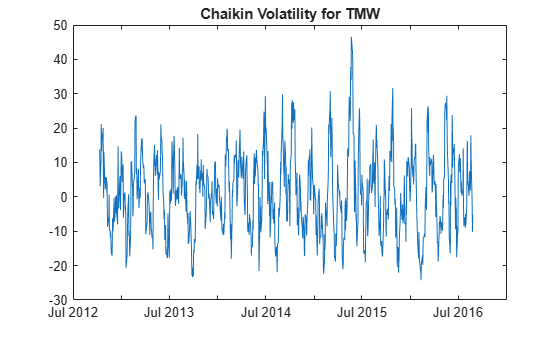Documentation

chaikvolat

Chaikin volatility

chaikvolat has been partially removed and will no longer accept a fints object (tsobj) argument. Use a matrix, timetable, or table instead for financial time series.

Use fts2timetable to convert a fints object to a timetable object.

Description

example

volatility = chaikvolat(Data) calculates the Chaikin volatility from a data series of high and low stock prices.

example

volatility = chaikvolat(___,Name,Value) adds optional name-value pair arguments.

Examples

collapse all

Load the file SimulatedStock.mat, which provides a timetable (TMW) for financial data for TMW stock.

volatility = chaikvolat(TMW,'NumPeriods',14,'WindowSize',14);
plot(volatility.Time,volatility.ChaikinVolatility)
title('Chaikin Volatility for TMW')Input Arguments

collapse all

Data with high, low, open, close information, specified as a vector, matrix, table, or timetable. For matrix input, Data is an M-by-2 matrix of high and low prices stored in the first and second columns. Timetables and tables with M rows must contain variables named 'High' and 'Low' (case insensitive).

Data Types: double | table | timetable

Name-Value Pair Arguments

Specify optional comma-separated pairs of Name,Value arguments. Name is the argument name and Value is the corresponding value. Name must appear inside quotes. You can specify several name and value pair arguments in any order as Name1,Value1,...,NameN,ValueN.

Example: volatility = chaikvolat(TMW,'NumPeriods',10,'WindowSize',10)

Period difference, specified as the comma-separated pair consisting of 'NumPeriods' and a scalar positive integer.

Data Types: double

Length of the exponential moving average in periods, specified as the comma-separated pair consisting of 'WindowSize' and a scalar positive integer.

Data Types: double

Output Arguments

collapse all

Chaikin volatility, returned with the same number of rows (M) and the same type (matrix, table, or timetable) as the input Data.

collapse all

Chaikin volatility

Chaikin volatility calculates the Chaikin's volatility from the series of high and low stock prices.

By default, Chaikin's volatility values are based on a 10-period exponential moving average and 10-period difference.

 Achelis, S. B. Technical Analysis from A to Z. Second Edition. McGraw-Hill, 1995, pp. 304–305.